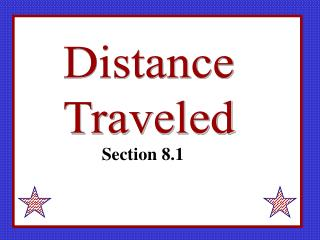DownloadDownload PresentationDistance Traveled

# Distance Traveled

Download Presentation## Distance Traveled

- - - - - - - - - - - - - - - - - - - - - - - - - - - E N D - - - - - - - - - - - - - - - - - - - - - - - - - - -
##### Presentation Transcript

1. Distance Traveled Section 8.1

2. Door My chair My desk If I start at my chair and walk to my desk and then back to my chair, what is my displacement? Total distance traveled? Displacement: 8+(-8)=0 Total distance: 8+8=16

3. Door My chair My desk If I start at my chair and walk to my desk and then to the door, what is my displacement? Total distance traveled? Displacement: 8+(-10)=-2 Total distance: 8+10=18 What is the significance of a positive or negative displacement? Is “total distance” ever negative?

4. Speed is the absolute value of velocity.

5. A honey bee makes several trips from the hive to a flower garden. The velocity graph is shown below. What is the total distance traveled by the bee? 700 feet 200ft 200ft 200ft 100ft

6. What is the displacement of the bee? 100 feet towards the hive 200ft 200ft -200ft -100ft

7. v (ft/sec) 14 4 7 t (sec) 5 10 A turtle starts 2 feet to the left of my mailbox. Where is the turtle at t=10? -4 What is the net distance traveled by the turtle? What is the total distance traveled by the turtle?

8. v (m/sec) 4 t (sec) 5 10 The velocity of a particle moving along the x-axis is given. -4 What is the displacement of the particle? What is the total distance traveled? If the particle started at x=3 when t=0, what is the position of the particle at 10 seconds?

9. To find the displacement (position shift) from the velocity function, we just integrate the function. The negative areas below the x-axis subtract from the total displacement. To find distance traveled we have to use absolute value. Find the roots of the velocity equation and integrate in pieces, just like when we found the area between a curve and the x-axis. (Take the absolute value of each integral.) Or you can use your calculator to integrate the absolute value of the velocity function.

10. WITH CALCULATOR An object moves along a coordinate line with the distance to the right considered positive. If the velocity of the object is given by over the time interval find the net and total distance traveled. Set up the problem if you wanted to know the net distance for

11. WITHOUT CALCULATOR An object moves along a coordinate line with the distance to the right considered positive. If the velocity of the object is given by over the time interval find the net and total distance traveled.

12. Find the net and total distance traveled by the moving object in the time interval given. Note that acceleration is given.

13. The velocity of a car was read at 10 second intervals and recorded in the table. Estimate the distance traveled by the car.

14. Homework p376 #1-15 all